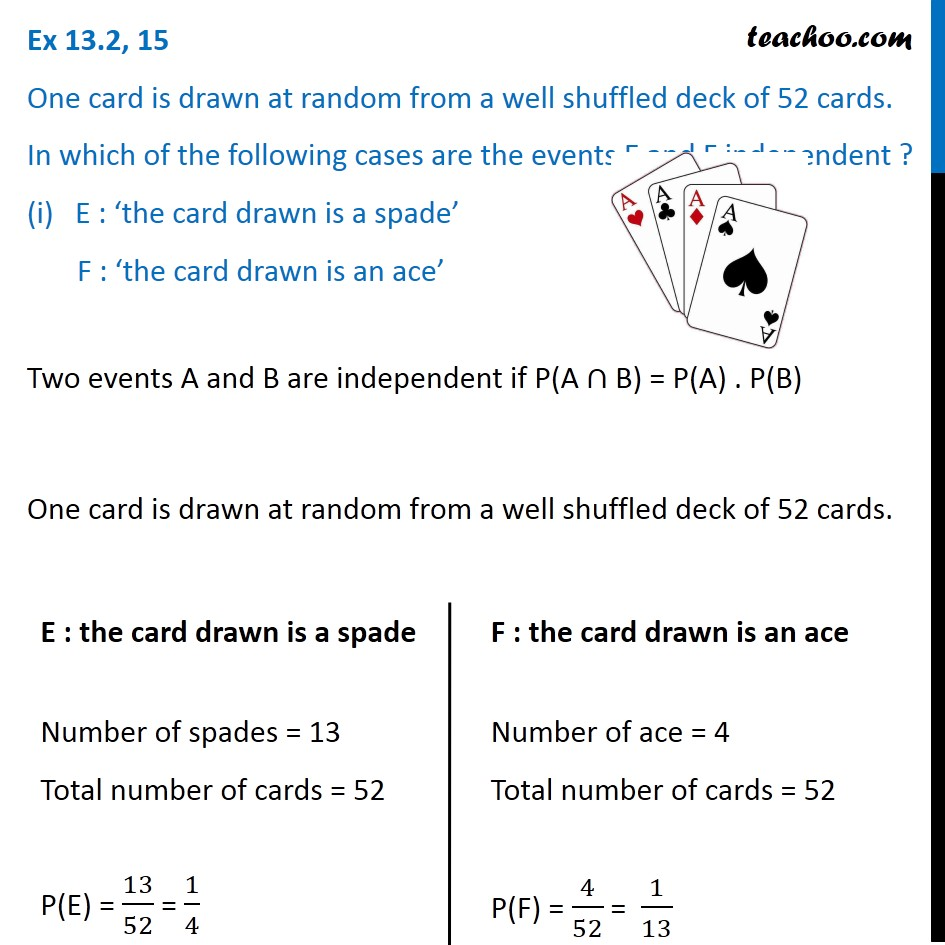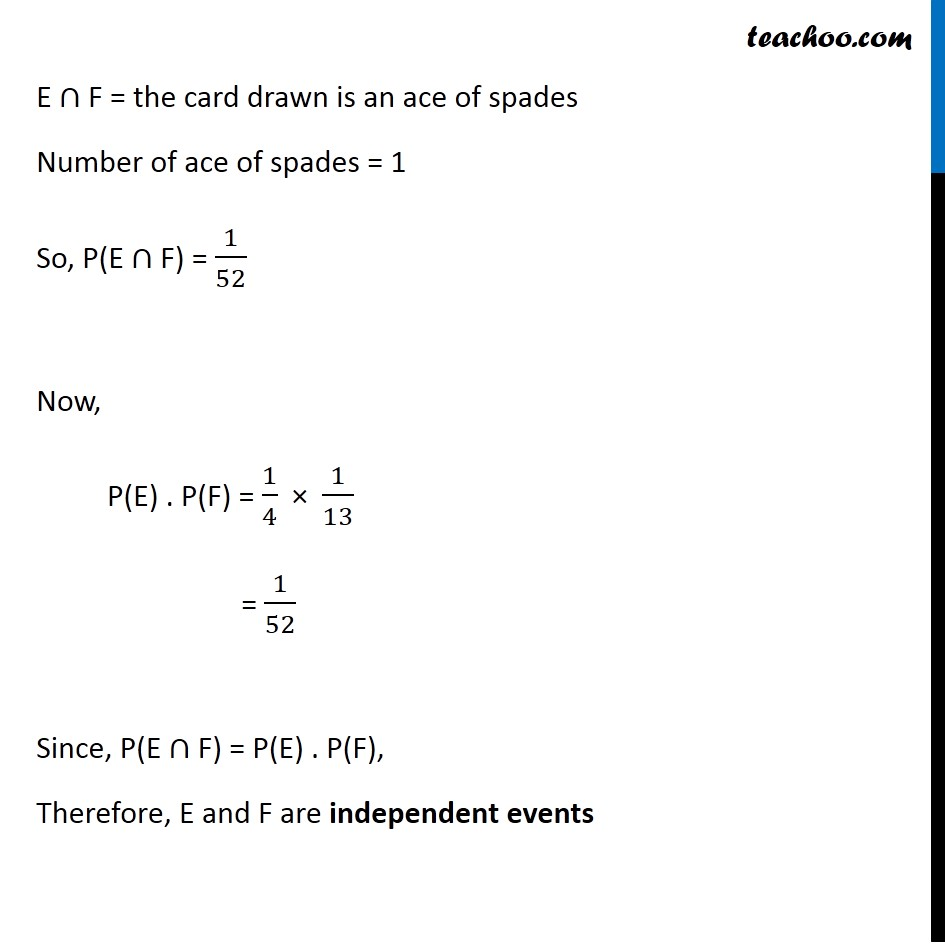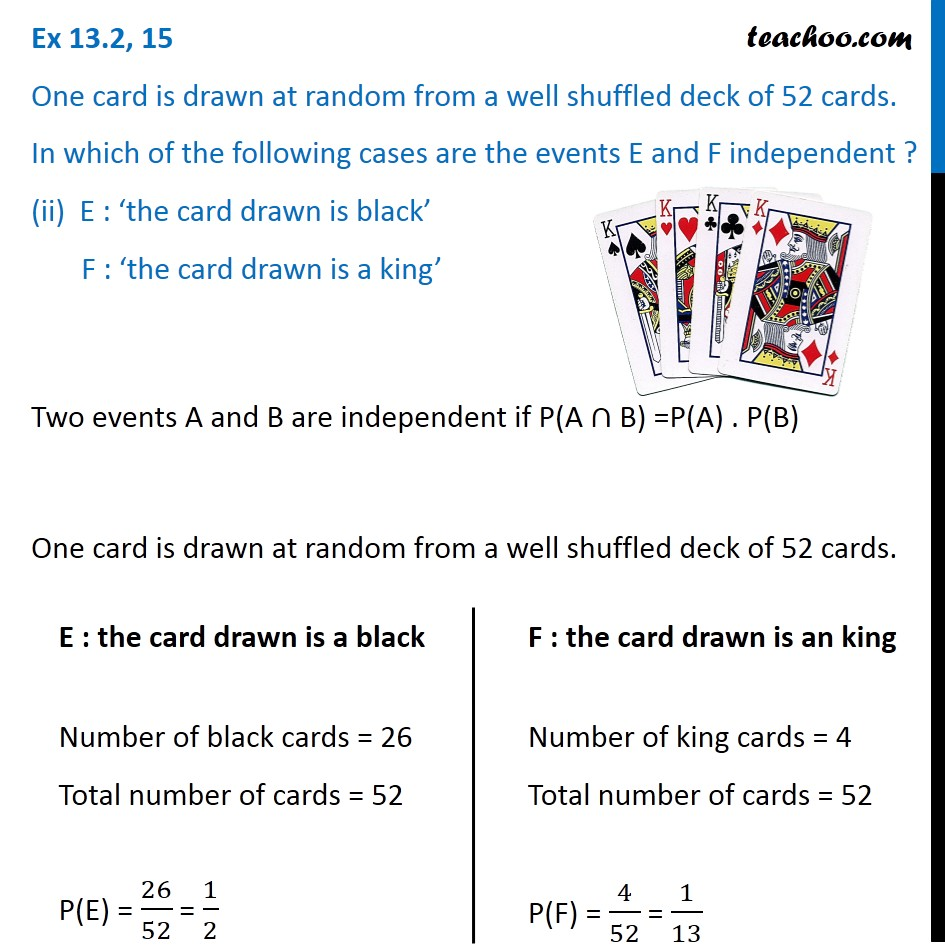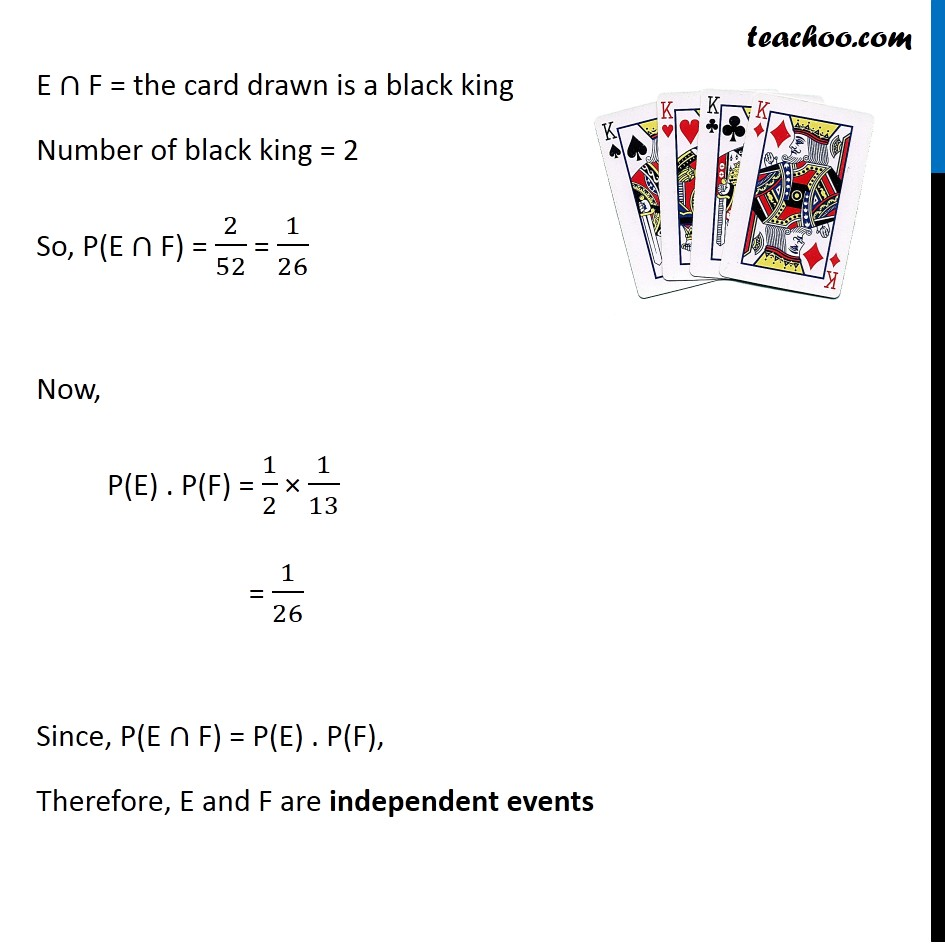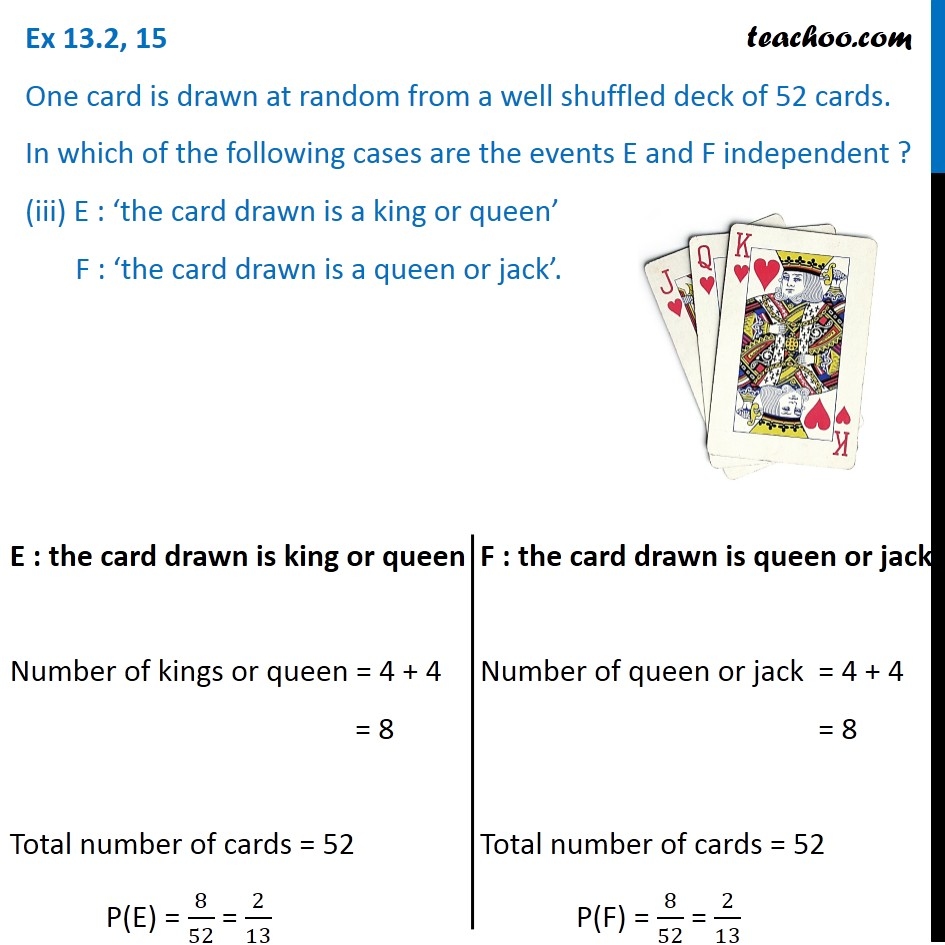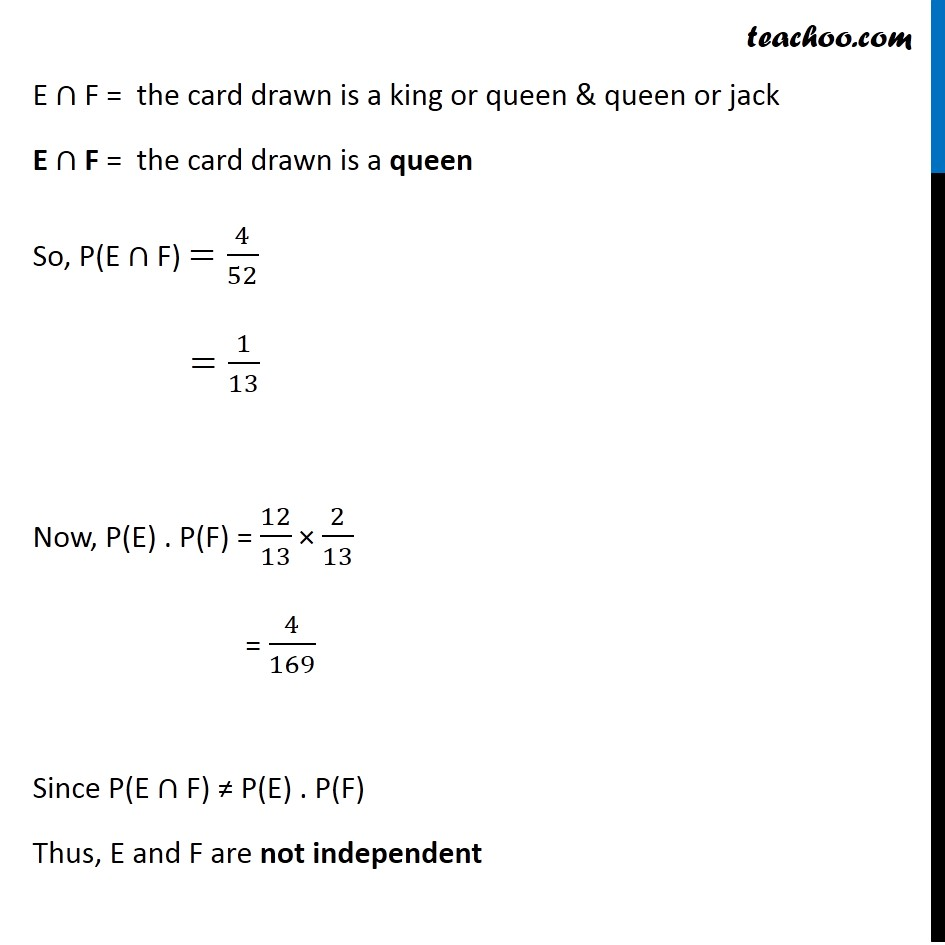Subscribe to our Youtube Channel - https://you.tube/teachoo

1. Chapter 13 Class 12 Probability
2. Serial order wise
3. Ex 13.2

Transcript

Ex 13.2, 15 One card is drawn at random from a well shuffled deck of 52 cards. In which of the following cases are the events E and F independent ? (i) E : ‘the card drawn is a spade’ F : ‘the card drawn is an ace’Two events A and B are independent if P(A ∩ B) = P(A) . P(B) One card is drawn at random from a well shuffled deck of 52 cards. E : the card drawn is a spade Number of spades = 13 Total number of cards = 52 P(E) = 13/52 = 1/4 F : the card drawn is an ace Number of ace = 4 Total number of cards = 52 P(F) = 4/52 = 1/13 E ∩ F = the card drawn is an ace of spades Number of ace of spades = 1 So, P(E ∩ F) = 1/52 Now, P(E) . P(F) = 1/4 × 1/13 = 1/52 Since, P(E ∩ F) = P(E) . P(F), Therefore, E and F are independent events Ex 13.2, 15 One card is drawn at random from a well shuffled deck of 52 cards. In which of the following cases are the events E and F independent ? (ii) E : ‘thea card drawn is a king’Two events A and B are independent if P(A ∩ B) =P(A) . P(B) One card is drawn at random from a well shuffled deck of 52 cards. E : the card drawn is a black Number of black cards = 26 Total number of cards = 52 P(E) = 26/52 = 1/2 F : the card drawn is an king Number of king cards = 4 Total number of cards = 52 P(F) = 4/52 = 1/13 E ∩ F = the card drawn is a black king Number of black king = 2 So, P(E ∩ F) = 2/52 = 1/26 Now, P(E) . P(F) = 1/2 × 1/13 = 1/26 Since, P(E ∩ F) = P(E) . P(F), Therefore, E and F are independent events Ex 13.2, 15 One card is drawn at random from a well shuffled deck of 52 cards. In which of the following cases are the events E and F independent ? (iii) E : ‘the card drawn is a king or queen’ F : ‘the card drawn is a queen or jack’.E : the card drawn is king or queen Number of kings or queen = 4 + 4 = 8 Total number of cards = 52 P(E) = 8/52 = 2/13 F : the card drawn is queen or jack Number of queen or jack = 4 + 4 = 8 Total number of cards = 52 P(F) = 8/52 = 2/13 E ∩ F = the card drawn is a king or queen & queen or jack E ∩ F = the card drawn is a queen So, P(E ∩ F) =4/52 =1/13 Now, P(E) . P(F) = 12/13 × 2/13 = 4/169 Since P(E ∩ F) ≠ "P(E) . P(F)" Thus, E and F are not independent

Ex 13.2